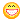The sending of the angles of rotation on the network Author Message
BabulesnikMember

 Post: #1 The sending of the angles of rotation on the network In the previous topic, I never got a solution to my problem. I really hope that someone will help me in this thread. I'll try to explain the essence of most of its problems. I'm creating a bowling game online.When I knocked the ball pins I need to transfer the position of the ball and pins through the network.Ie the angles of the axes x, y, z and the position (Vec).The problem is in the rotation angles. If I give the angles: Code: `Vec angles = ItemsBowling.matrix().angles();` And turn the object of a neighbor on the angles: Code: `ItemsNeighbor.matrix().setRotateXY(angle.x, angle.y).setRotateZ(angle.z).move(pos)` I am not getting the correct rotation:( How to get angles and rotate neighbor EE::Item on this angles ?
02-11-2012 07:29 PM
EsenthelAdministratorPost: #2 RE: The sending of the angles of rotation on the network Try ItemsNeighbor.matrix(Matrix().setRotateZ(angle.z).rotateXY(angle.x, angle.y).move(pos));
02-12-2012 04:17 PM
BabulesnikMember

 Post: #3 RE: The sending of the angles of rotation on the network (02-12-2012 04:17 PM)Esenthel Wrote:  Try ItemsNeighbor.matrix(Matrix().setRotateZ(angle.z).rotateXY(angle.x, angle.y).move(pos)); Thank you very much!!! Now everything is working fine02-12-2012 09:03 PM
 « Next Oldest | Next Newest »